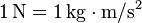# Newton (unit) facts for kids

Kids Encyclopedia Facts

The newton (symbol: N) is the SI unit of force. It is named after Sir Isaac Newton because of his work on classical mechanics. A newton is how much force is required to make a mass of one kilogram accelerate at a rate of one metre per second squared.$1\, \mathrm{N}=1\, \mathrm{kg} \cdot \mathrm{m} / \mathrm{s}^{2}$
• 1 N is the force of Earth's gravity on an apple with a mass of about 102 g.
• On the Earth's surface, a mass of 1 kg pushes on its support with an average force of 9.8 N.
• 1 Newton is equal to the amount of force required to accelerate an object at a rate of 1 metre per second, every second. One newton is the force needed to accelerate one kilogram of mass at the rate of one metre per second squared in direction of the applied force.
• The US Customary Unit of force is the pound (symbol: lbf). 1 pound is equal to 4.44822 newtons.

In 1946, Conférence Générale des Poids et Mesures (CGPM) set the unit of force in the MKS system of units to be the amount needed to accelerate 1 kilogram of mass at the rate of 1 metre per second each second. In 1948, the CGPM adopted the name "newton" for this force. The MKS system then became the blueprint for today's SI International System of Units. That made the newton the standard unit of force.

This SI unit is named after Isaac Newton. As with every International System of Units (SI) unit named for a person, the first letter of its symbol is upper case (N). However, when an SI unit is spelled out in English, it should always begin with a lower case letter (newton)—except in a situation where any word in that position would be capitalized, such as at the beginning of a sentence or in material using title case. Note that "degree Celsius" conforms to this rule because the "d" is lowercase.— Based on The International System of Units, section 5.2.

Newton's second law of motion states that F = ma, where F is the force applied, m is the mass of the object receiving the force, and a is the acceleration of the object. The newton is therefore:Newton (unit) Facts for Kids. Kiddle Encyclopedia.